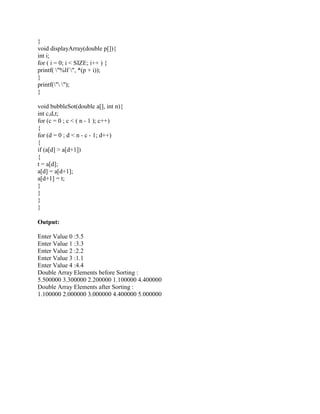AnúncioPróximos SlideSharesData struture lab
Carregando em ... 3
1 de 3
Anúncio

### Please use printf- This is C programming- Design a program that will i.docx

1. Please use printf. This is C programming. Design a program that will input numeric data type double into an array. Do this function by designing a function called inputData . Then the program should sort the data and display the whole content of the array. Sort the array by using bubble sort ( use the qsort function or /you can Google that ) and design a separate function (call it displayArray) to display the contents of the array. Please make sure that it is double type and use printf, I asked before this question but the answer was incorrect, hopefully this time the answer would be correct. Thanks Solution Sort.C #include <stdio.h> #define SIZE 5 double* inputData(); void displayArray(double p[]); void bubbleSot(double a[], int n); int main() { double *a = inputData(); printf("Double Array Elements before Sorting : "); displayArray(a); bubbleSot(a, SIZE); printf("Double Array Elements after Sorting : "); displayArray(a); return 0; } double* inputData(){ static double arr[SIZE]; int i; for(i=0; i<SIZE; i++){ printf("Enter Value %d :",i); scanf("%lf", &arr[i]); } return arr;
2. } void displayArray(double p[]){ int i; for ( i = 0; i < SIZE; i++ ) { printf( "%lf ", *(p + i)); } printf(" "); } void bubbleSot(double a[], int n){ int c,d,t; for (c = 0 ; c < ( n - 1 ); c++) { for (d = 0 ; d < n - c - 1; d++) { if (a[d] > a[d+1]) { t = a[d]; a[d] = a[d+1]; a[d+1] = t; } } } } Output: Enter Value 0 :5.5 Enter Value 1 :3.3 Enter Value 2 :2.2 Enter Value 3 :1.1 Enter Value 4 :4.4 Double Array Elements before Sorting : 5.500000 3.300000 2.200000 1.100000 4.400000 Double Array Elements after Sorting : 1.100000 2.000000 3.000000 4.400000 5.000000
Anúncio